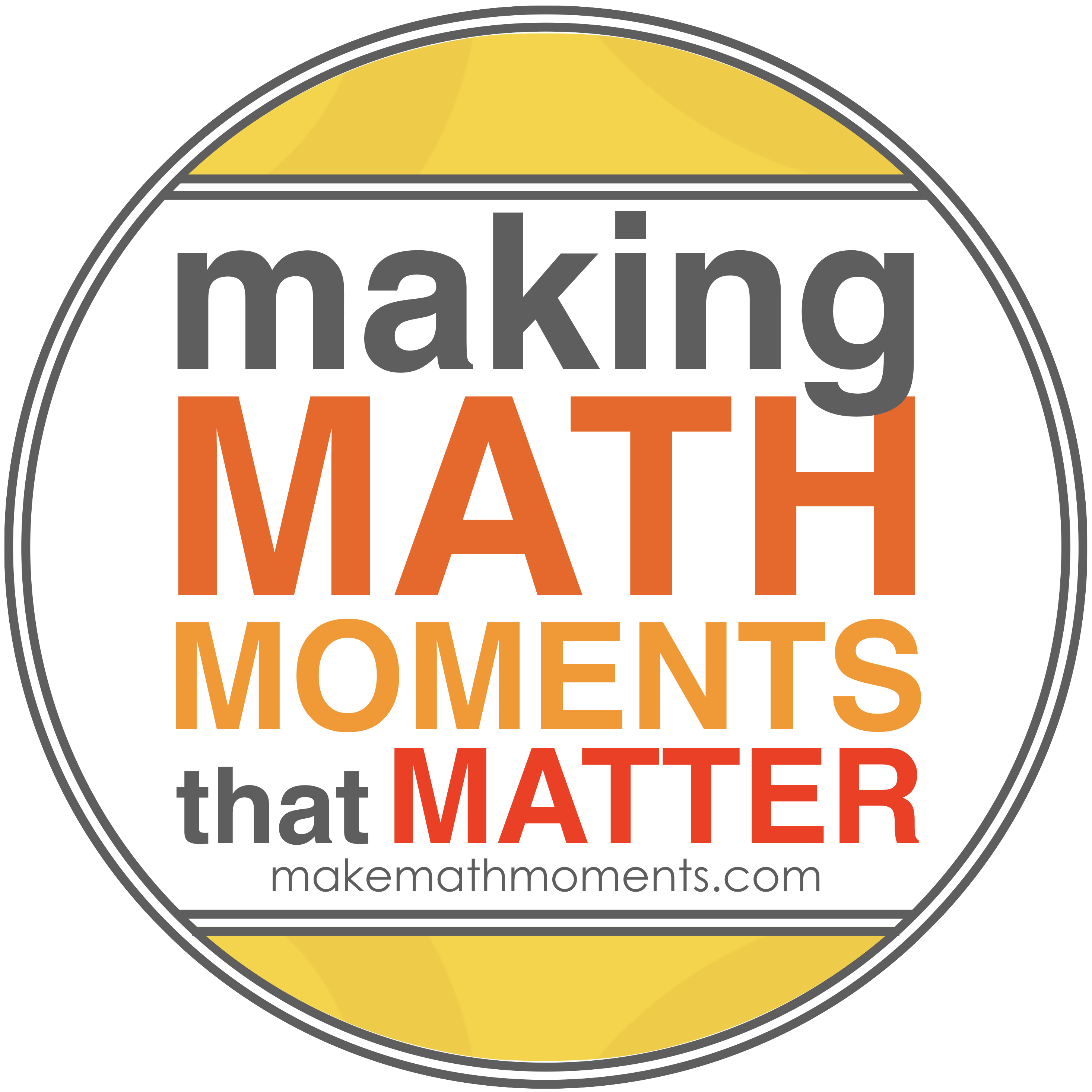# 3U

 COURSE NAME: Functions COURSE CODE:  MCR 3U1 MINISTRY GUIDELINE:  The Ontario Curriculum, Grades 11                                                Mathematics, 2006 Revised LEVEL OF DIFFICULTY:  University Prep CREDIT VALUE:  1 PREREQUISITE:    Principles of Mathematics, Grade 10, Academic TEXTBOOK(s):  Functions 11, McGraw-Hill Ryerson

Course Description:

This course introduces the mathematical concept of the function by extending students’ experiences with linear and quadratic relations. Students will investigate properties of discrete and continuous functions, including trigonometric and exponential functions; represent functions numerically, algebraically, and graphically; solve problems involving applications of functions; investigate inverse functions; and develop facility in determining equivalent algebraic expressions. Students will reason mathematically and communicate their thinking as they solve multi-step problems.

Overall Learning Expectations:

By the end of this course, students will:

• demonstrate an understanding of functions, their representations, and their inverses, and make connections between the algebraic and graphical representations of functions using transformations;
• determine the zeros and the maximum or minimum of a quadratic function, and solve problems involving quadratic functions, including those arising from real-world applications;
• demonstrate an understanding of equivalence as it relates to simplifying polynomial, radical, and rational expressions;
• evaluate powers with rational exponents, simplify expressions containing exponents, and describe properties of exponential functions represented in a variety of ways;
• make connections between the numeric, graphical, and algebraic representations of exponential functions;
• identify and represent exponential functions, and solve problems involving exponential functions, including those arising from real-world applications;
• demonstrate an understanding of recursive sequences, represent recursive sequences in a variety of ways, and make connections to Pascal’s triangle;
• demonstrate an understanding of the relationships involved in arithmetic and geometric sequences and series, and solve related problems;
• make connections between sequences, series, and financial applications, and solve problems involving compound interest and ordinary annuities;
• determine the values of the trigonometric ratios for angles less than 360º; prove simple trigonometric identities; and solve problems using the primary trigonometric ratios, the sine law, and the cosine law;
• demonstrate an understanding of periodic relationships and sinusoidal functions, and make connections between the numeric, graphical, and algebraic representations of sinusoidal functions;
• identify and represent sinusoidal functions, and solve problems involving sinusoidal functions, including those arising from real-world applications.

-2-

Evaluation:

Term Work                                                                                        70%

Knowledge / Understanding                                    30 %

Inquiry / Thinking                                                20 %

Communication                                                20 %

Application / Making Connections                        30 %

Assessment activities during the course will be comprised of a variety of methods and strategies.  (eg.  Assignments, projects, tests, journals, performances, conferences, daily work, etc.)

Final Evaluation                                                                              30%

The final evaluation will consist of, but is not limited to, a final examination, written during the examination schedule.

Classroom Rules:

Students are expected to follow these rules so that mathematics education can be successful.

a) BE ON TIME

b) COME PREPARED FOR CLASS

c) PARTICIPATE IN CLASS – Keep good notes while the lesson is being taught, pay

d) RESPECT THE RIGHTS OF OTHERS – Work quietly and co-operate so as not to

disturb other students who are trying to learn.

e) CATCH UP ON ANY WORK MISSED – When returning from an absence, catch up

on missed work as quickly as possible.  This is your responsibility.

f) COMPLETE ANY HOMEWORK ASSIGNED – If work is not completed in class,

complete it before the next class so that you may benefit from the discussions based on

the homework.

g) REMAIN IN YOUR DESK until the teacher dismisses the class.

h) FOLLOW ESTABLISHED SCHOOL ROUTINES AND BEHAVIOUR CODES – see

student handbook.

Learning Materials Required:

• Textbook
• Notebook – 3 ring binder with paper
• Pencil, eraser, ruler
• Scientific calculator
• Student Handbook## CONTACT ME